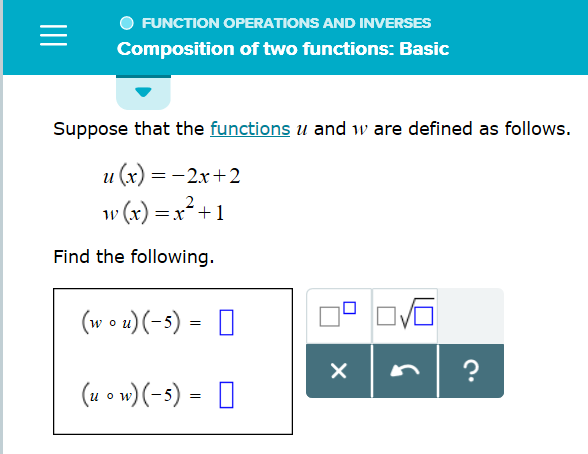# FUNCTION OPERATIONS AND INVERSES Composition of two functions: Basic Suppose that the functions u and w are defined as follows. u (x)2x+2 ()x2+1 1V Find the following. (w u)(-5) O ? (u o w)(-5) = X

Questionhelp_outlineImage TranscriptioncloseFUNCTION OPERATIONS AND INVERSES Composition of two functions: Basic Suppose that the functions u and w are defined as follows. u (x)2x+2 ()x2+1 1V Find the following. (w u)(-5) O ? (u o w)(-5) = X fullscreen

### Want to see the step-by-step answer?